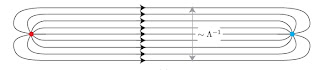Tuesday, 3 February 2009

Quirks

Back in the old days life was so easy. Everybody knew that the right theory at the TeV scale was the MSSM who in turn was a compactification of the heterotic string theory. One could lead a quite life picking colors for the mSUGRA parameter space. This perfect world began to crumble at the turn of century. At first, the alternative models had the same objective as supersymmetry in mind: to solve the hierarchy problem and explain the lightness of the Higgs boson. But soon the rules got relaxed and everything became allowed. Recently, there has been a lot theoretical activity related to models motivated not by naturalness of electroweak symmetry breaking but rather by exotic collider signatures that are not covered by standard experimental searches. I guess the first example of such unmotivated model was split supersymmetry; unparticles and hidden valleys are more recent examples of this trend. Quirks, advertised in this paper by Junhai Kang and Markus Luty, is the latest addition in that list.

Imagine there is a hidden sector in the form of an SU(N) gauge theory. In addition, the hiddensector contains a quark (vector-like fermion in the fundamental representation of SU(N)) with mass m. Another mass parameter characterizing the gauge theory is the confinement scale \$\Lambda\$ - the analog of the QCD scale \$\Lambda_{QCD}\$ - where the hidden gluons and quarks become confined into glueballs, mesons and baryons. So far it looks standard. What distinguishes quirks from the familiar QCD-like dynamics is that the quark mass is much larger than the condensation scale:
\$m \gg \Lambda\$
and there are no other quarks that are lighter.

Models with such a heavy quark have pretty weird, quirky properties. To understand why, recall what happens in the usual QCD when a quark-antiquark pair is produced. The two quarks would like to fly apart from the collision point but, because of the nature of strong interactions, there is a color flux tube forming between them. This flux tube can be thought of as a string connecting the two quarks - that's what they call confinement. The energy stored in the string is proportional to the distance between the quarks, and to the string tension which is of the order of the QCD confinement scale \$\Lambda_{QCD}\$. In QCD, the string energy per unit length is large enough to produce a new quark-antiquark pair out of the vacuum. In consequence, the QCD string snaps. The end result is a lot of hadronic junk flying along the directions of the initial quarks that materialize as jets in a detector.

In the case of quirks, the energy of the string per unit length is far too small to rip a pair of new quirks out of the vacuum. Thus, the quirky string does not break. Furthermore, quirks cannot decay to the glueballs, even though the latter are much lighter, because of conserved quantum numbers. Once produced, two quirks are chained together by the string and oscillate back and forth. The typical length of the string is given \$m/\Lambda^2\$. Plugging in \$m = 1\$ TeV (so that we can produce quirks at the LHC) and \$\Lambda\$ between 100 GeV and 100 eV (why not), the length of the string varies from \$10^{-17}\$m (microscopic) to 10m (detector size!). That's why the experimenters at the LHC wear protective helmets: one may get hit anytime by the loose end of the string.In order to produce quirks at the LHC, the quirks must be coupled to the Standard Model somehow. The simplest thing to assume is to give quirks the usual electric or color charge, although there are other possibilities. The search strategy also depends on the model assumptions. One phenomenologically important factor is the typical length of the string connecting the quirks.If the string is macroscopic, that is larger than a millimeter (corresponding to \$\Lambda <10 a="" and="" annihilates="" annihilation="" antiquirk="" are="" argue="" back="" be="" because="" bound="" br="" brings="" broad.="" brown="" but="" by="" can="" cannot="" case="" collider="" completely="" context="" control="" could="" curvature="" decay="" details="" detector.="" difference="" different="" discovery.="" distribution="" easy="" employed="" event="" exerted="" exotic="" field="" for="" force="" if="" in="" invariant="" is="" kev="" less="" long-lived="" magnetic="" mass="" may="" mean="" microscopic="" millimeter="" muck="" not="" observe="" of="" one="" ordinary="" original="" pair="" particles.="" process="" products="" quirk-antiquirk="" quirk="" quirks="" quite="" rate="" resolve="" search="" separate="" shorter="" signatures="" similar="" so="" spectacular.="" spectacular="" state="" strategy="" string="" strings="" suppressed.="" surrounding="" than="" that="" the="" them="" then="" theoretically="" these="" this="" thus="" to="" together.="" tracks="" two="" ultimately="" varies="" very="" which="" would="">
More details in the paper. See also slides from a recent talk at CERN.

1 comment:

dir said...

it is an interesting and clearly written post on quirks. in last few months, i listened two talks relevant to quirks. i always have had difficulties in understanding the claim that the typical length of the string is given \$m/\Lambda^2\$. my non-perturbative qcd picture makes me feel that the string length is always \$1/\Lambda\$, irrelevant to m. although m is larger and heavy quarks do not decay, when one stretches the heavy quark pair, the energy you use transforms into glue-ball emission, instead of making the string longer. namely one sees many glue-balls coming out while the length of the string does not change much.